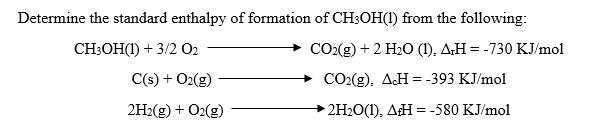# Determine the standard enthalpy of formation of CHsOH() from the following: CH3OH(I) + 3/2 02 CO2(g) + 2 H20 (I), Δ,Η =-730 KJ/mol C(s) O2(gCO2g) AcH-393 KJ/mol 2H2(g) + 02(g)2 2H201), AH 580 KJ/mol

Questionhelp_outlineImage TranscriptioncloseDetermine the standard enthalpy of formation of CHsOH() from the following: CH3OH(I) + 3/2 02 CO2(g) + 2 H20 (I), Δ,Η =-730 KJ/mol C(s) O2(gCO2g) AcH-393 KJ/mol 2H2(g) + 02(g)2 2H201), AH 580 KJ/mol fullscreen

### Want to see the step-by-step answer?